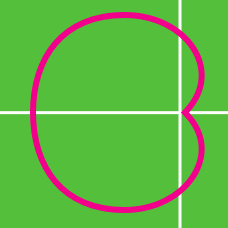Calculus

# Polar Coordinates Introduction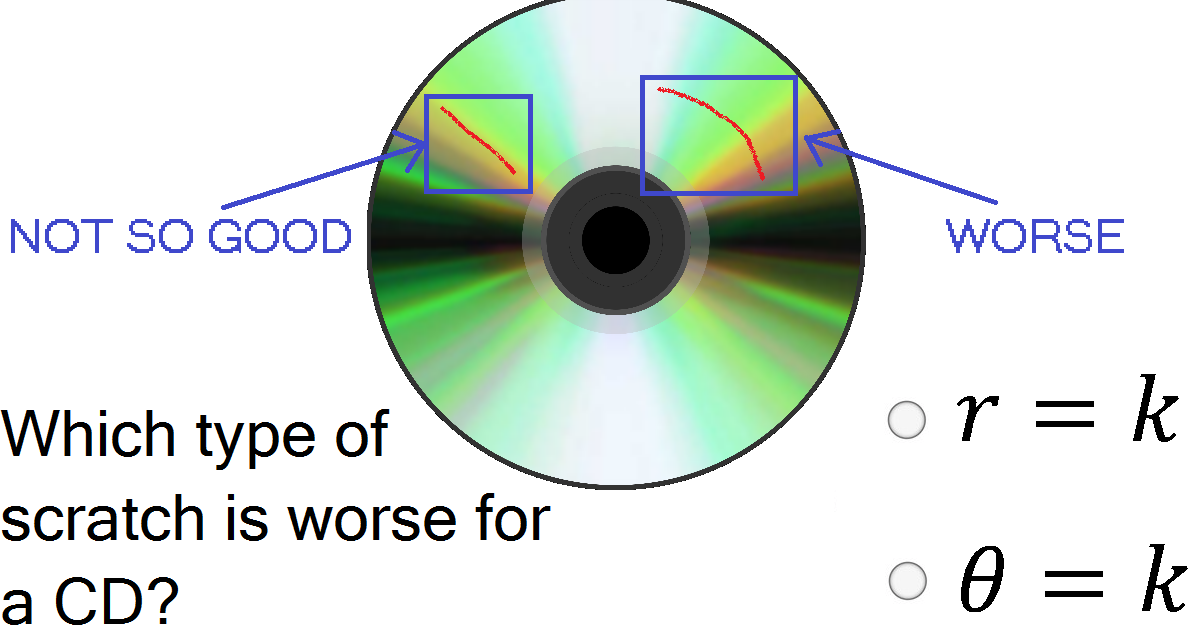If the CD is placed on a polar coordinate system with the origin in the center of the hole in the center of the CD, which type of equation does the picture indicate is the worse type of equation for a scratch to approximate?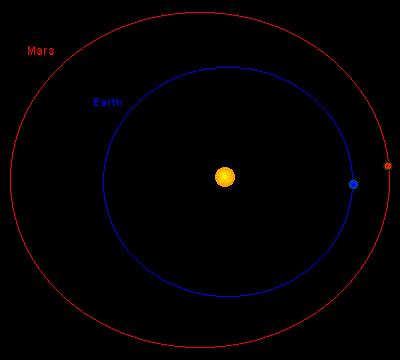The Earth and Mars' orbits are approximated by elliptical functions. When placed on the same coordinate system with the Sun at the center, the two orbits are approximated by the polar equations

$A: r= \frac{1 - (.03)^2}{1+.03\cos\theta}$

and

$B: r= 1.52\left(\frac{1 - (.09)^2}{1+.09\cos\theta}\right).$

Which is the equation for Earth's orbit?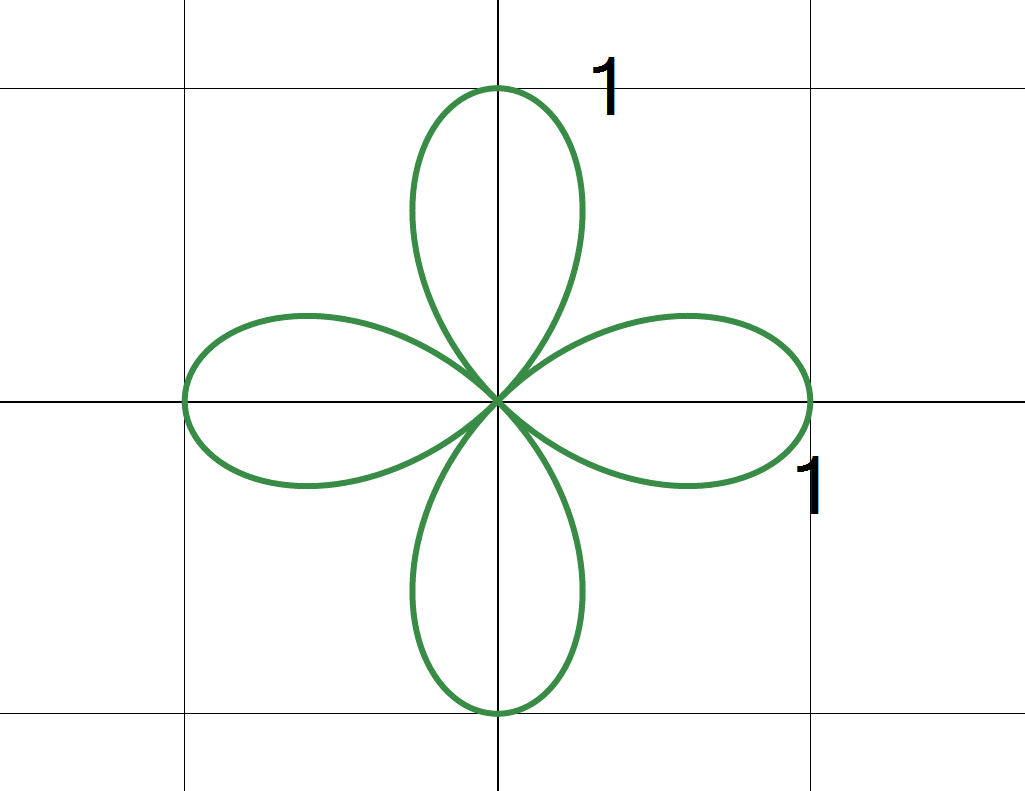The equation

$(x^2 + y^2)^3 = (x^2 - y^2)^2,$

whose graph is shown, describes a Quadrifolium. What is its equation in polar coordinates?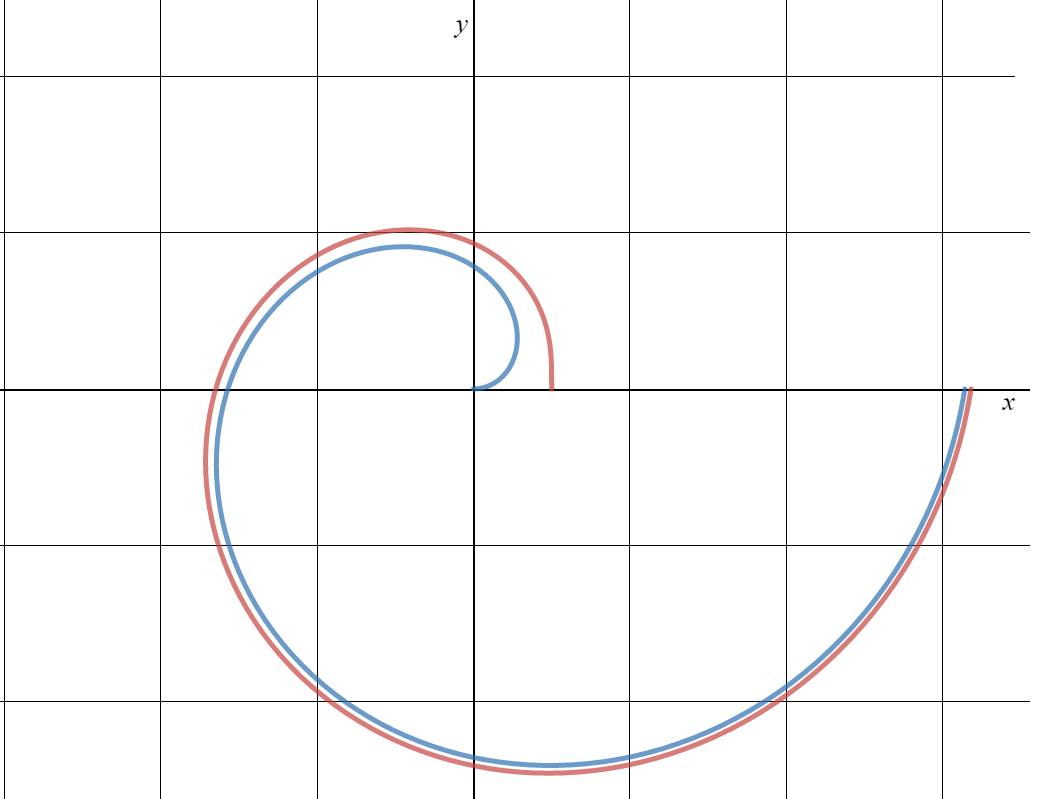The picture shows, for $0 \leq \theta \leq 2\pi,$ the graph of an Archimedian Spiral with equation $r = \theta,$ and an involute of a circle having equation $r = \sqrt{1 + \theta^2}.$

Which is the graph of the Archimedean Spiral?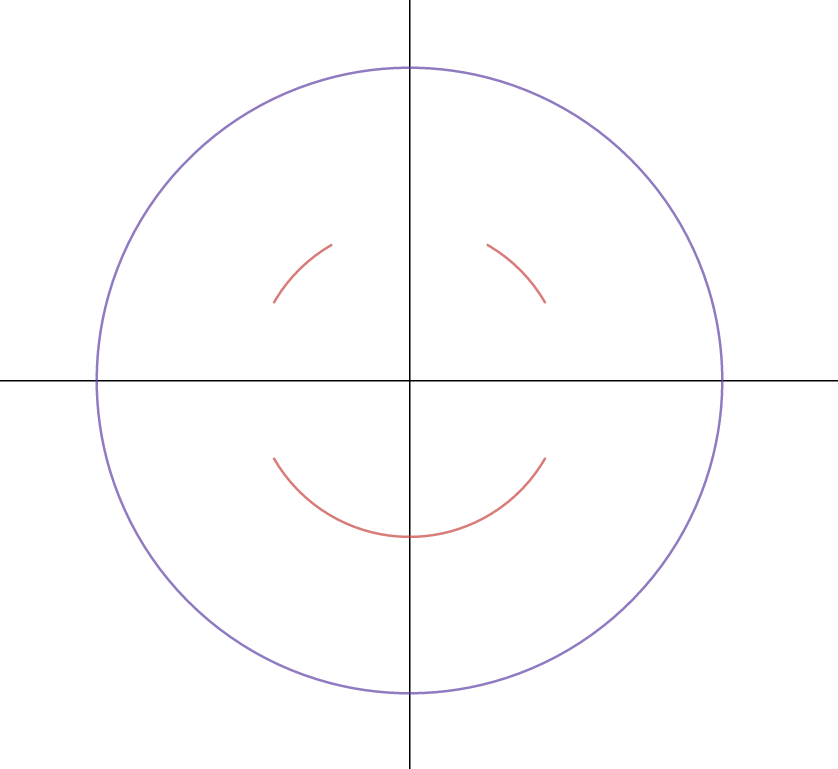Chance had some time on his hands and drew this picture using the polar graphing feature of his graphing program. Which answer choice best describes the mouth of this picture?

×